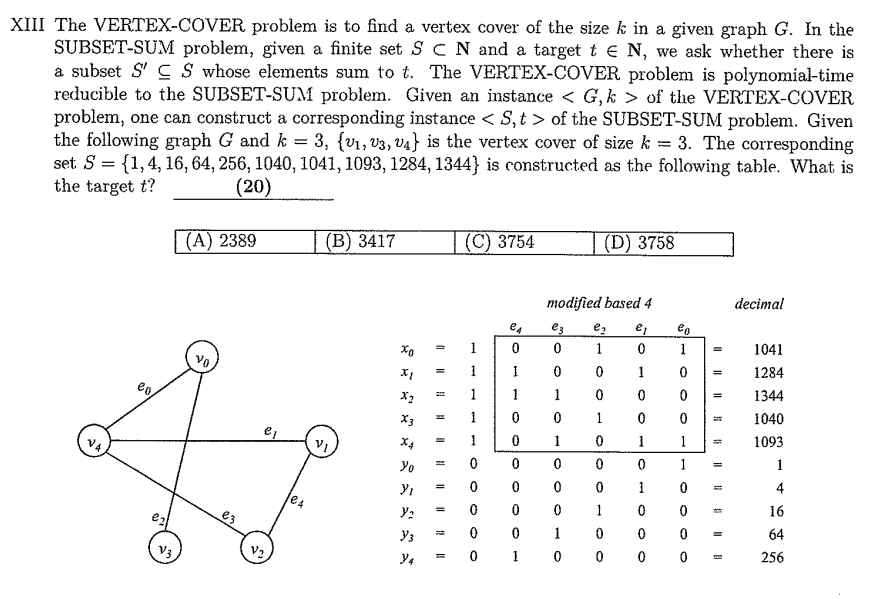# 台大資工所110碩士班軟體參考解答$I.$ When CAN the worst case of Quicksort occur? _____(1)_____
$\small \quad C_{1}:$ Quick Sort where leftmost (or rightmost) element is always chosen as pivot
$\small \quad C_{2}:$ leftmost element is chosen as pivot and array is already sorted in SAME order
$\small \quad C_{3}:$ leftmost element is chosen as pivot and array is already sorted in REVERSE order
$$\small (A) \, C_{1} \quad (B) \, C_{2} \quad (C) \, C_{1} \, \text{and} \, C_{2} \quad (D) \, C_{2} \, \text{and} \, C_{3}$$

$II.$ A typical dynamic programming example is the snake sequence. Given a grid of numbers, find maximum length snake sequence and print it. A snake sequence is made up of adjacent numbers in the grid such that for each number, the number on the right or the number below it is $\small +1$ or $\small -1$ its value. For example, if you are at location $\small (x, \, y)$ in the grid, you can either move right $\small i.e. (x+1, \, y)$ if that number is $\small \pm 1$ or move down $\small i.e. (x, \, y+1)$ if that number is $\small \pm 1$. The length of a snake sequence is defined as the number of moves. Given the following grid (A matrix with $\small M$ rows and $\small N$ columns),

\small \qquad \qquad \begin{aligned} &9, \, 6, \, 5, \, 2 \\ & 8, \, 7, \, 6, \, 5 \\ &7, \, 3, \, 1, \, 6 \\ &1, \, 1, \, 1, \, 7 \end{aligned}

$\small (a)$ The maximum LENGTH of snake sequence is _____(2)_____
$$\small (A) \, 6 \quad (B) \, 7 \quad (C) \, 5 \quad (D) \, 4$$
$\small (b)$ Time complexity of above solution is _____(3)_____
$$\small (A) \, O(M \times N) \quad (B) \, O(M^2) \quad (C) \, O(N^2) \quad (D) \, O(M)$$
$\small (c)$ The last number of the maximum-length snake sequence is _____(4)_____
$$\small (A) \, 9(0, \, 0) \quad (B) \, 6(3, \, 2) \quad (C) \, 7(3, \, 3) \quad (D) \, 1(2, \, 3) \quad (E) \, 7(0, \, 2)$$
$\small (d)$ The third number of the maximum-length snake sequence is _____(5)_____
$$\small (A) \, 7(1, \, 1) \quad (B) \, 7(0, \, 2) \quad (C) \, 3(1, \, 2) \quad (D) \, 6(2, \, 1) \quad (E) \, 8(0, \, 1)$$

$III.$ For questions with sequences, we constantly split the problem into a number of sub-problems that are smaller instances of the same problem, and we try to solve the smaller problem recursively. We called this type of algorithm _____(6)_____
$$\small (A) \, \text{Dynamic programming} \quad (B) \, \text{Divide and conquer} \quad (C) \, \text{Greedy algorithms} \quad (D) \, \text{Quicksort}$$

$IV.$ There is a large enough array $\small A$ with indices $\small p$ and $\small q$ which are initialized by $\small 0$, and functions $\small f(x)$ and $\small g()$ are defined as follows. Array $\small A$ and indices $\small p$ and $\small q$ can only be accessed in the functions $\small f(x)$ and $\small g()$.

\small \qquad \qquad \begin{aligned} &f(x) \\ &\cdot \, p \leftarrow p + 1 \\ &\cdot \, A[p] \leftarrow x \\ &g() \\ &\cdot \, x \leftarrow A[p] \\ &\cdot \, p \leftarrow p – 1 \\ &\cdot \, \text{return} x \end{aligned}

$\small (a)$ Which data structure is operated by those functions? _____(7)_____
$$\small (A) \, \text{Queue} \quad (B) \, \text{Stack} \quad (C) \, \text{Hash} \quad (D) \, \text{Heap}$$
$\small (b)$ If functions $\small f(x)$ and $\small g()$ are changed as following:

\small \qquad \qquad \begin{aligned} &f(x) \\ &\cdot \, A[q] \leftarrow x \\ &\cdot \, q \leftarrow q + 1 \\ &g() \\ &\cdot \, x \leftarrow A[p] \\ &\cdot \, p \leftarrow p + 1 \\ &\cdot \, \text{return} x \end{aligned}

Which data structure is operated by those functions? _____(8)_____
$$\small (A) \, \text{Queue} \quad (B) \, \text{Stack} \quad (C) \, \text{Hash} \quad (D) \, \text{Heap}$$

(a) C
(b) A

(a) C
(b) D

(a) B
(b) BGrandpa

1.周辰表示:
1.Grandpa表示: